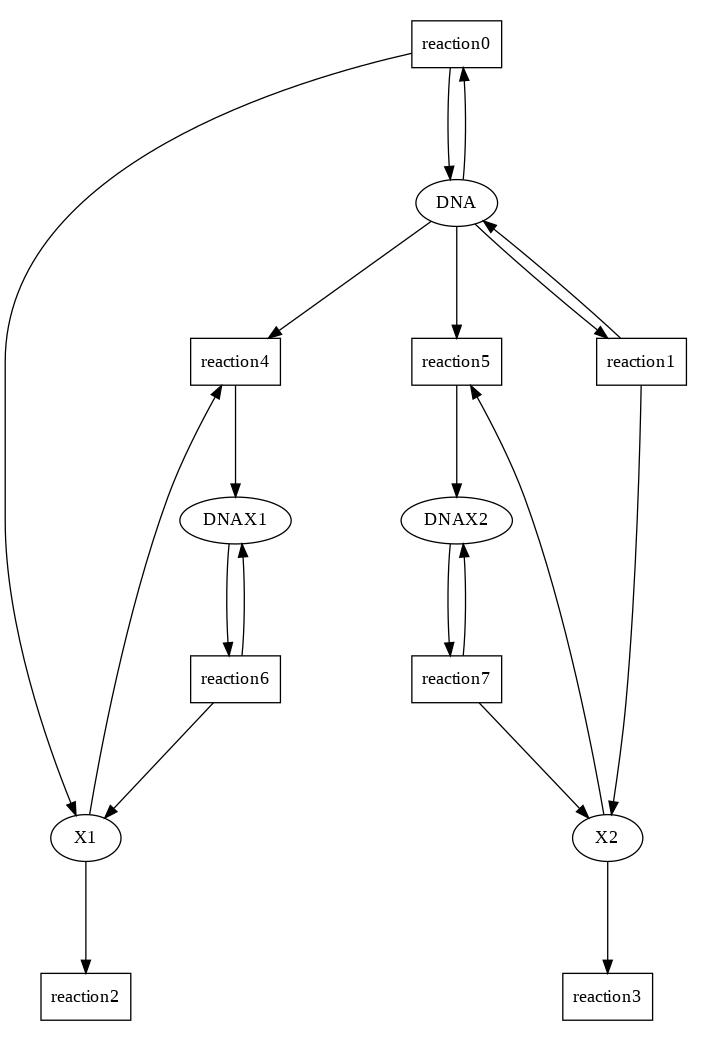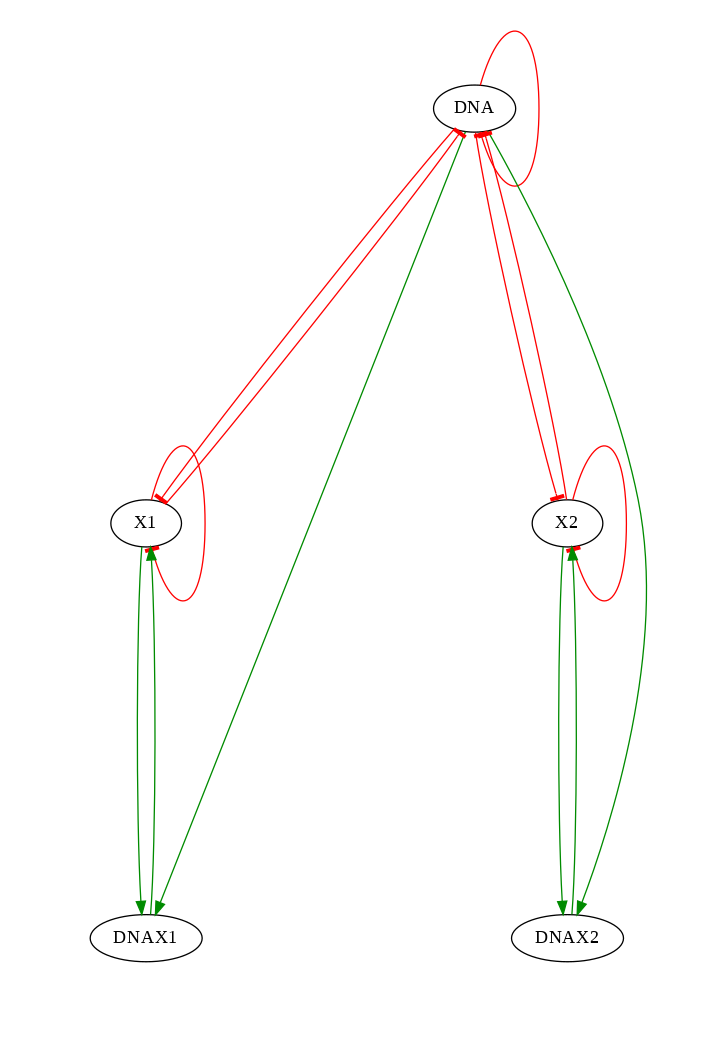# TD4: Toggle Switch¶

1. Competing syntheses with cooperativity
2. Stochastic semantics: toggle switch
3. Continuous semantics: first-order approximation of the mean stochastic behavior

F. Fages 25 Jan 2019

Exclusive Switch (Loinger et al., Phy. Rev. E, 2007) Two genes with common promoter Slow or fast binding to the promoter with rate constant kLet us first consider a model with irreversible binding to the promotor

In :
MA(s1) for DNA => DNA + X1.

In :
MA(s2) for DNA => DNA + X2.

In :
MA(d1) for X1 => _.

In :
MA(d2) for X2 => _.

In :
MA(b1) for DNA + X1 => DNAX1.

In :
MA(b2) for DNA + X2 => DNAX2.

In :
MA(sb1) for DNAX1 => DNAX1 + X1.

In :
MA(sb2) for DNAX2 => DNAX2 + X2.

In :
parameter(s1=1, d1=1, b1=1, sb1=10).
parameter(s2=1, d2=1, b2=1, sb2=10).

In :
present(DNA, d). parameter(d=0.01).

In :
draw_reactions.In :
draw_influences.In :
search_conservations.

Out:
DNA+DNAX1+DNAX2
1 complex invariant(s)


## Stochastic semantics¶

In :
option(time:200, method:ssa).

In :
numerical_simulation. plot.


## Slow vs fast binding rates of the promotors X1 X2¶

• Fast binding rates create cooperativity and a toggle switch stochastic behavior
• Slow binding rates delete cooperativity and create oscillating behavior
In :
%slider b1 b2 sb1 sb2


## Boolean semantics¶

• possible stability of DNAX1 or DNAX2 or no free DNA
• possible boolean oscillations for X1 and X2
• (oscillations are not expressible in Computation Tree Logic CTL, an over-approximation formula is used)
In :
generate_ctl_not.

Out:
reachable(stable('DNAX1'))
reachable(stable('DNAX2'))
reachable(stable(not'DNA'))
reachable(steady('DNA'))
reachable(steady('X1'))
reachable(steady('X2'))
reachable(steady(not'X1'))
reachable(steady(not'X2'))
oscil('X1')
oscil('X2')

In :
expand_ctl(oscil(f)).

Out:
EU(not f,f/\EU(f,not f/\EU(not f,f/\EU(f,not f/\EU(not f,f)))))/\EG(EF(f)/\EF(not f))

In :
list_stable_states.

Out:
[DNA-0,DNAX1-0,DNAX2-0,X1-0,X2-0]


## Continuous semantics¶

• Deterministic semantics
• First-order approximation of the mean stochastic behavior
• Same picture for slow and fast binding rates
In :
parameter(b1=1, b2=1). option(method:bsimp).

In :
numerical_simulation. plot.

In :
plot(show:X2, against:X1).

In :
%slider b1 b2

In :
plot(show:X2, against:X1).


# Questions¶

### 1) add unbinding reactions from the promotor with kinetic parameters u1, u2¶

In :
MA(ub1) for DNAX1 => DNA + X1.

In :
MA(ub2) for DNAX2 => DNA + X2.

In :
parameter(ub1=2, ub2=2).
present(DNA, d). parameter(d=0.01).


### 2) Do stochastic simulations and explain the differences with the previous stochastic behavior¶

use the cells below for stochastic simulations, possibly Boolean model-checking, and

explain your answer here

...

In :
option(time:200, method:ssa).

In :
numerical_simulation. plot.


As expected, the bistability phenomenon is far less pronounced, since the promoters can unbind, so having one of the $X_i$ binding the DNA is not sufficient anymore to keep producing $X_i$ forever (in other words, the DNAXi states are not stable anymore).

### 3) Give parameter values to b1, b2, sb1, sb2 to recover similar stochastic behavior as before¶

use the cells below for stochastic simulations with sliders and

give your answer here

...

In :
%slider b1 b2 sb1 sb2 ub1 ub2


The bistability phenomenon reappaears when $b_1, b_2 >> ub_1, ub_2$ (as expected: when binding is way more likely than unbinding, resulting in a situation similar to the without-unbinding one).

For example, with:

Parameter Value
$b_1, b_2$ 100
$ub_1, ub_2$ 1
$sb_1, sb_2$ 1000